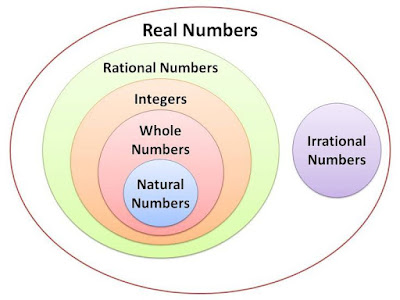## Monday, May 11, 2020

### Number Systems: Naturals, Integers, Rationals, Irrationals, Reals

Number Systems: Naturals, Integers, Rationals, Irrationals, Reals

## Number Systems: Naturals, Integers, Rationals, Irrationals, Reals

We use numbers in our day to day life. They are often called numerals. Without numbers, we cannot do counting of things, date, time, money, etc. Sometimes these numbers are used for measurement and sometimes they are used for labelling.
There are different types of numbers in Maths, which we learn.We will discuss all the types here in this article.

### Introduction to number system

The collection of numbers is called the Number system. These numbers are of different types such as natural numbers, whole numbers, integers, rational numbers and irrational numbers. Let us see the table below to understand with the examples.

 Natural Numbers N 1, 2, 3, 4, 5, …… Whole Numbers W 0,1, 2, 3, 4, 5…. Integers Z …., -3, -2, -1, 0, 1, 2, 3, … Rational Numbers Q p/q form, where p and q are integers and q is not zero. Irrational Numbers Which can’t be represented as rational numbers

### Types of Numbers

• Natural Numbers: Natural numbers are known as counting numbers that contain the positive integers from 1 to infinity. The set of natural numbers is denoted as “N” and it includes N = {1, 2, 3, 4, 5, ……….}
• Whole Numbers: Whole numbers are known as non-negative integers and it does not include any fractional or decimal part. It is denoted as “W” and the set of whole numbers includes W = {0,1, 2, 3, 4, 5, ……….}
• Integers: Integers are the set of all whole numbers but it includes a negative set of natural numbers also. “Z” represents integers and the set of integers are Z = { -3, -2, -1, 0, 1, 2, 3}
• Real Numbers: All the positive and negative integers, fractional and decimal numbers without imaginary numbers are called real numbers. It is represented by the symbol “R”.
• Rational Numbers: Any number that can be written as a ratio of one number over another number is written as rational numbers. This means that any number that can be written in the form of p/q. The symbol “Q” represents the rational number.
• Irrational Numbers: The number that cannot be expressed as the ratio of one over another is known as irrational numbers and it is represented by the symbol ”P”.
• Complex Numbers: The number that can be written in the form of a+bi where “a and b” are the real number and “i” is an imaginary number is known as complex numbers “C”.
• Imaginary Numbers: The imaginary numbers are the complex numbers that can be written in the form of the product of a real number and the imaginary unit “i”.Number System

Please do not paste any url....

## Featured Post